# Reciprocal Calculator

To find the reciprocal, choose the type of the number then enter the values in the reciprocal calculator.

Give Us Feedback

The reciprocal calculator is a math tool that finds the reciprocal of different types of numbers. It can find the reciprocal of:

To see the calculation process step-by-step, click “Show More after calculating the reciprocal.”

## What is a reciprocal?

The reciprocal of a number is calculated by taking the same number under division to 1. Such as the reciprocal of number 13 is 1 over 13 (1/13).

## How to find the reciprocal?

You can use the reciprocal fraction calculator or solve it by yourself. To learn how to find the reciprocal of different numbers, continue reading.

### Reciprocal of decimal numbers:

It is easy to find the reciprocal of numbers and integers but when they have a decimal point, it is a little different.

Example

Find the reciprocal of 1.34.

Solution:

Step 1: Make it a numerator by placing one in the fraction.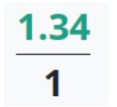Step 2: Swap the numerator and denominator.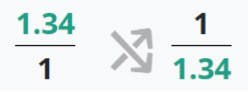Step 3: Convert the decimal to fraction.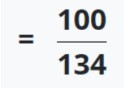Step 4: Solve.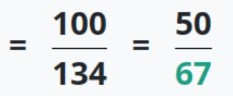You can solve it more to find the decimal point value.

### Reciprocal of fractions:

In the previous example, you can see how to find the reciprocal of a simple fraction. Just swap places of the values and that’s it. For mixed fractions, see the example ahead.

Example

What is the reciprocal of195?

Solution:

Step 1: Convert the mixed fraction to a simple fraction.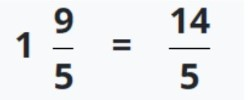Step 2: Invert the fraction.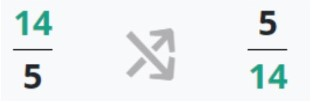If you want then you can solve to get a decimal number value in step 3.

### Math Tools